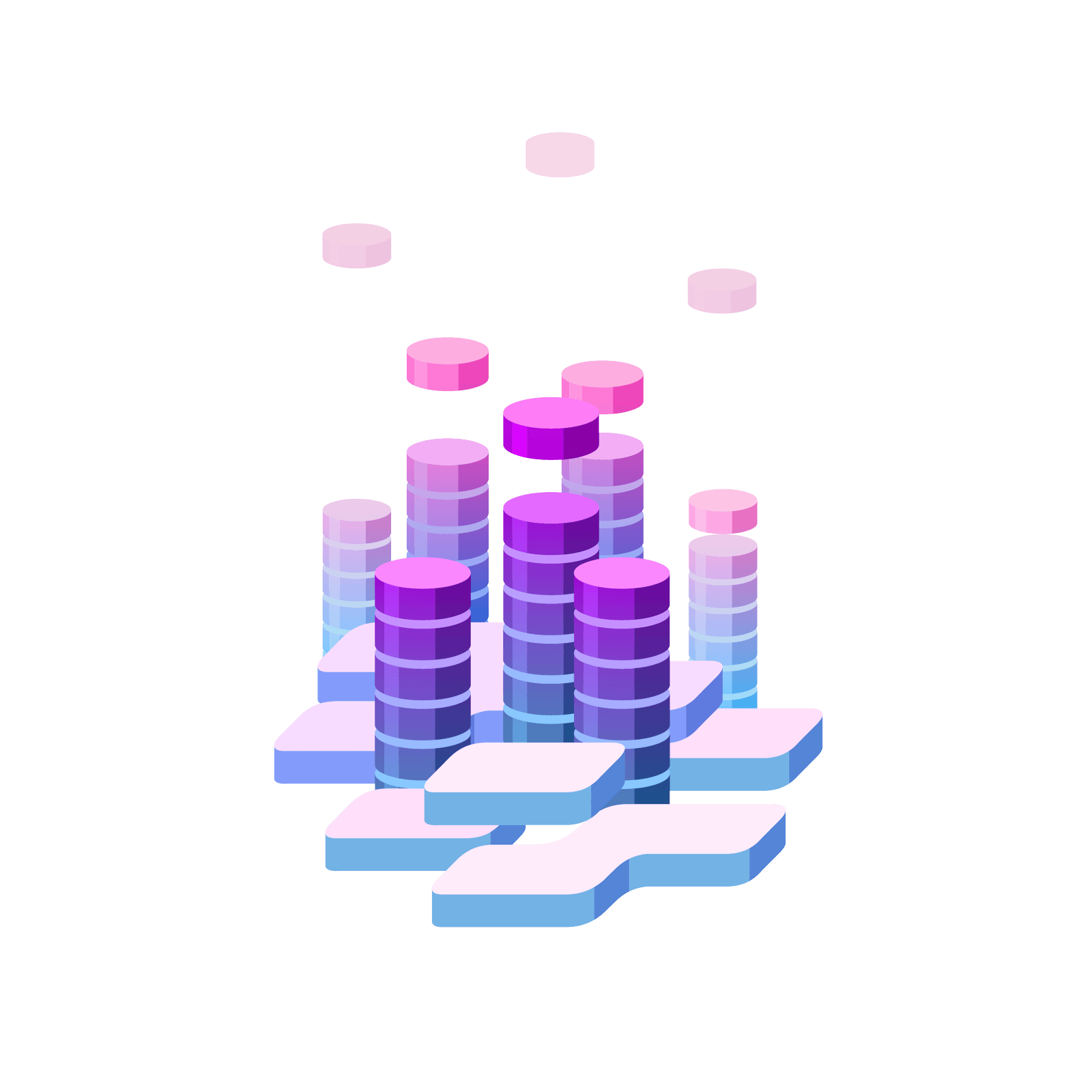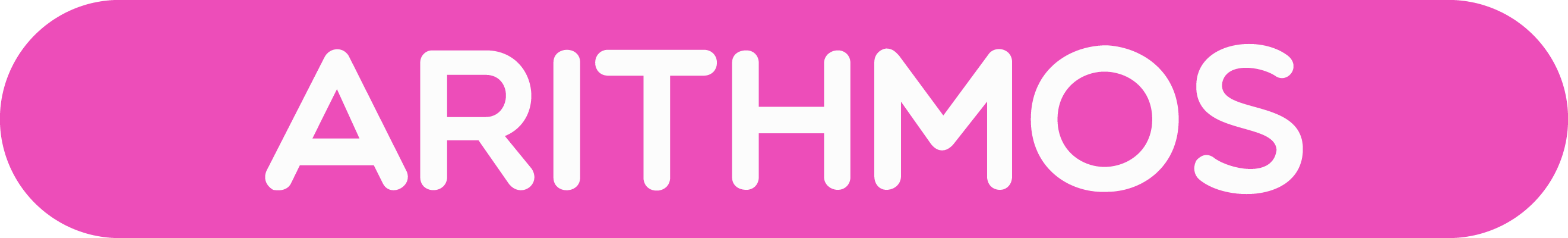06 285 000# Station  HLong MultiplicationIs it possible to do, say, $37\times 23$, with dots and boxes?

### Question 1

Here we are being asked to multiply three tens by $23$ and seven ones by $23$.

If we are good with our multiples of $23$, we will realize that this must give must give $69$ tens (since $3\times 23 = 69$) and $161$ ones (since $7\times 23 = 161$).

The answer is thus $69|161$.

With explosions this becomes $851$.### Question 2

When Suzzy thought about $37\times 23$ for a little while, she eventually drew the following diagram:She then said that $37\times 23 = 6 | 23 | 21 = 8 | 3 | 21 = 851$.

Can you work out what Suzzy was thinking?

What diagram do you think Suzzy might draw for $236\times 34$ (and what answer will she get from it)?

### Question 3

Using Suzzy’s approach do $37\times 23$ and $23\times 37$ give the same answer? Is it obvious as you go through the process that they will?

Do $236\times 34$ and $34\times 236$ give the same answer in Suzzy’s approach?

### Question 4

Here’s another fun way to think about multiplication. Let’s do it in a $1\leftarrow 2$ machine this time.

Let’s work out $13\times 3$.

Start by representing thirteen in a $1\leftarrow 2$ machine.### Question 5

We’re being asked to triple everything. So each dot we see is to be replaced with three dots.And now we can do some explosions to see the answer $39$ appear (which is $100111$ in the $1\leftarrow 2$ machine).

Try this on the machine above and show that the answer $100111$ would appear if we had the sixth box in the machine.

Alternatively, we can notice that three dots in a $1\leftarrow 2$ machine actually look like this.So we can replace each dot in our picture of $13$ instead by one dot and one dot one place to the left. (I’ve added some colour to the picture to help.)Now with less explosions to do, we see the answer $100111$ appear.

Try this too on the machine above.You can either play with some of the optional stations below or go to the next island!

### Let's Go Wild!